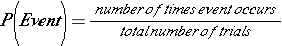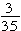# Experimental Probability

In this lesson, you will learn how to estimate the probability of an event by using experimental probability. Experimental probability is the ratio of the total number of times the favorable outcome occurs to the total number of trials, or times the experiment is performed. Experimental probability is helpful when you want to make predictions about something. It is important to note that the more trials you have (the more times you perform the experiment), the more accurate your prediction is likely to be. The experimental probability can be written as### ExampleLucian used a spinner for his experiment. He created a table to display the results.

 Number on spinner 1 2 3 4 5 6 7 Times Landed 10 4 6 3 7 3 2

Based on the data, what is the probability that the spinner will land on 6 on the next spin?

1. Find the number of trials: 10 + 4 + 6 + 3 + 7 + 3 + 2 = 35

2. Find the number of times the event happened – The spinner landed on 6 three times.

3. Write the probability as a fraction in simplest form.The probability of the spinner landing on 6 the next spin is. This can be written as a decimal or a percent: .086 or 8.6%.

### ExampleCourtney made 15 baskets out of 25 free throw attempts. What is the experimental probability that she will make her next shot? P(basket)

1. Find the number of trials – 25 (attempts)

3. Write the probability as a fraction, decimal, and percent. P(basket) = 3/5, 0.60, 60%Watch the video to see more examples of experimental probability.

## Experimental Probability Practice

Find each experimental probability. Write your answer as a fraction, a decimal, and a percent.

Make sure to answer with this format: 1/4; 0.25; 25%

1. A high school quarterback completes 10 of 16 passes. What is the experimental probability that his next pass will be incomplete?
2. Julie watches students entering the school one morning. She sees that 8 out of 25 are wearing boots. What is the experimental probability that the next student that Julie observes will be wearing boots?
3. Angela makes 12 out of 16 foul shots. What is the experimental probability that she will miss her next foul shot?
4. A researcher surveys 80 people to determine whether they will vote for Ford Gravitt for mayor. 42 out of 80 say ‘yes’. What is the experimental probability that the next person surveyed will say he or she plans to vote for Ford Gravitt?
5. An official police radar measured the speed of 40 cars. The radar shows that 14 of the 40 cars are going faster than the posted speed limit. What is the experimental probability that the next car will be traveling at or below the speed limit?
6. Nate hits an archery target 7 out of 20 times. What is the experimental probability that he will hit the target on his next try?

(source)